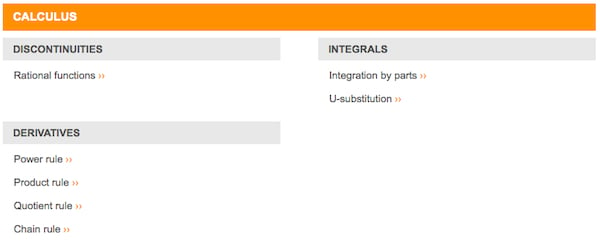# Using Wolfram|Alpha to Understand Mathematics

Mathematics: it's a subject intimidating to most, but painless for Wolfram|Alpha, the internet's most powerful computational knowledge engine. We've already looked at practical ways you can use Wolfram|Alpha in your daily life, but if you're in school or work in fields that require advanced mathematics, Wolfram|Alpha's roots in Mathematica are infinitely valuable.

The flaw in many online mathematics programs is that they may solve the problem, but inhibit further learning and understanding of mathematical concepts. This is not the case for Wolfram|Alpha. In this tutorial, I'll show you how to make the most out of Wolfram|Alpha's mathematical knowledge so that you may garner a better understanding of math.

Note: Some of the features showcased in this tutorial require a subscription to Wolfram|Alpha's Pro service, which costs $6.99/month for regular users and$4.99/month for students.

## Working Out a Solution

Type the math problem you want to solve into the search bar, and press Enter to submit the problem. Wolfram|Alpha will solve it in a matter of seconds, spitting out the answer at the top of the page.

Tip: Pro users can upload a photo or file of a math problem directly to Wolfram|Alpha. Wolfram|Alpha will solve the problem it detects in the file, thus saving time by eliminating the need to type in the problem.

But there's only so much getting the answer to the problem can do for you. This is where Wolfram|Alpha takes problem solving to the next level.

Pro subscribers are able to view step-by-step solutions to nearly any problem by pressing the Step-by-step solution button in the top-right corner of the solution field. Step-by-step solutions walk through each part of the solution process, complete with annotations about specific rules that were used to come to the solution. This allows you to gain a better understanding of how to approach similar problems.

Tip: Save the walkthroughs by clicking the Data download button in the Step-by-step solution window so it may be easily accessed later.

## Learning Mathematical Concepts

Perhaps Wolfram|Alpha used a specific rule in solving the problem that you are unfamiliar with. To get more information about this rule, simply search it in Wolfram|Alpha. The search will pull general information about the rule in order to explain it.

Wolfram|Alpha searches may also be conducted on broader mathematical concepts. To do so, search the concept you want to learn more about. You may need to add the term mathematical definition to find the proper definition of the concept you are searching.

For broader mathematical concepts, Wolfram|Alpha typically provides both a basic and detailed definition of the concept, as well as related topics. In the bottom corner of the definition field, there may be a More information button. Clicking this button redirects you to the Wolfram MathWorld page for the respective concept. Wolfram MathWorld is Wolfram's mathematics resource and provides very detailed explanations of mathematical concepts and rules, usually complete with proofs and visuals to better explain the concept.

Tip: Even if the More information button is unavailable from the Wolfram|Alpha page, it may still be searched in MathWorld for a more detailed explanation.

## Visualizing Problems

Especially as you dabble into more advanced mathematics, visualizing a problem may be the only way to truly understand what is happening. Luckily, Wolfram|Alpha has powerful visualization tools to aid in understanding a problem.

Every user is able to view plots and 3D models of functions. To do so, simply search plot [function] or [3D shape] image, and the proper visual will be generated.

Pro users can make use of Wolfram|Alpha's CDF interactivity, which allows for a more hands-on approach to interpreting data. All of the plots and images that nonsubscribers can see can be made interactive by clicking the Enable interactivity button in the bottom right corner of the Image or Plots section. Interactivity on these graphs allow you to manipulate the functions as you please.

Wolfram|Alpha also has animated plots for problems that involve time and motion, which are especially helpful when trying to wrap your head around a tough physics problem or parametric function.

## Extra Practice

Pro subscribers to Wolfram|Alpha have access to Wolfram Problem Generator, which churns out practice problems in six different mathematical areas: arithmetic, algebra, calculus, statistics, number theory, and linear algebra.

Within each of the subject areas, there is a selection of topics which may be practiced. While there isn't yet practice problems for quite a few concepts (such as series practice for Calculus), most of the basic concepts in each of the subject areas are covered.While many mathematical topics are covered in the problem generator, there are a few that are missing.

After choosing the topic you want to practice, select the difficulty of the problem in the Difficulty drop-down menu. There are the options of Beginner, Intermediate, and Advanced, but even the beginner questions are relatively difficult upon entering more advanced mathematics.

The Problem Generator affords three chances to answer the question correctly. Upon entering an incorrect answer, you may view a hint. After the second incorrect answer, you may view the Step-by-Step solution for the problem. You can skip problems by pressing the Skip this question button at the bottom right hand corner of the screen.

Tip: Monitor your progress by checking the History section at the bottom of the page. This will monitor how many questions you've been getting right or wrong.

You can also create a worksheet of problems by clicking the Random set or From history button, found beneath Generate a printable problem sheet under the problem generator. Clicking Random set will create a 20-question multiple choice worksheet complete with an answer key. The From history button will create a worksheet of problems you've previously attempted, complete with an answer sheet as well. Both options allow you to download the worksheet as a PDF to be edited or printed.